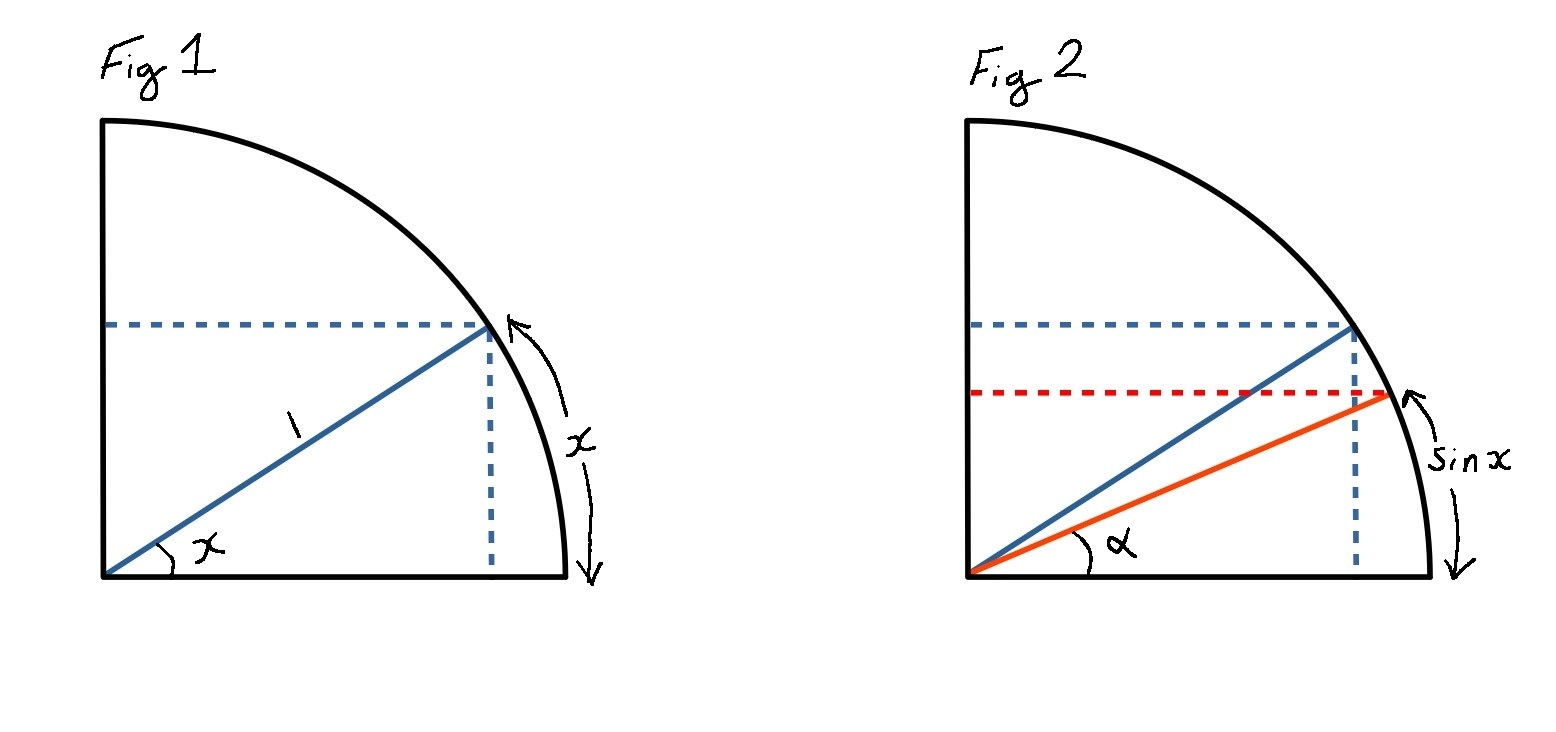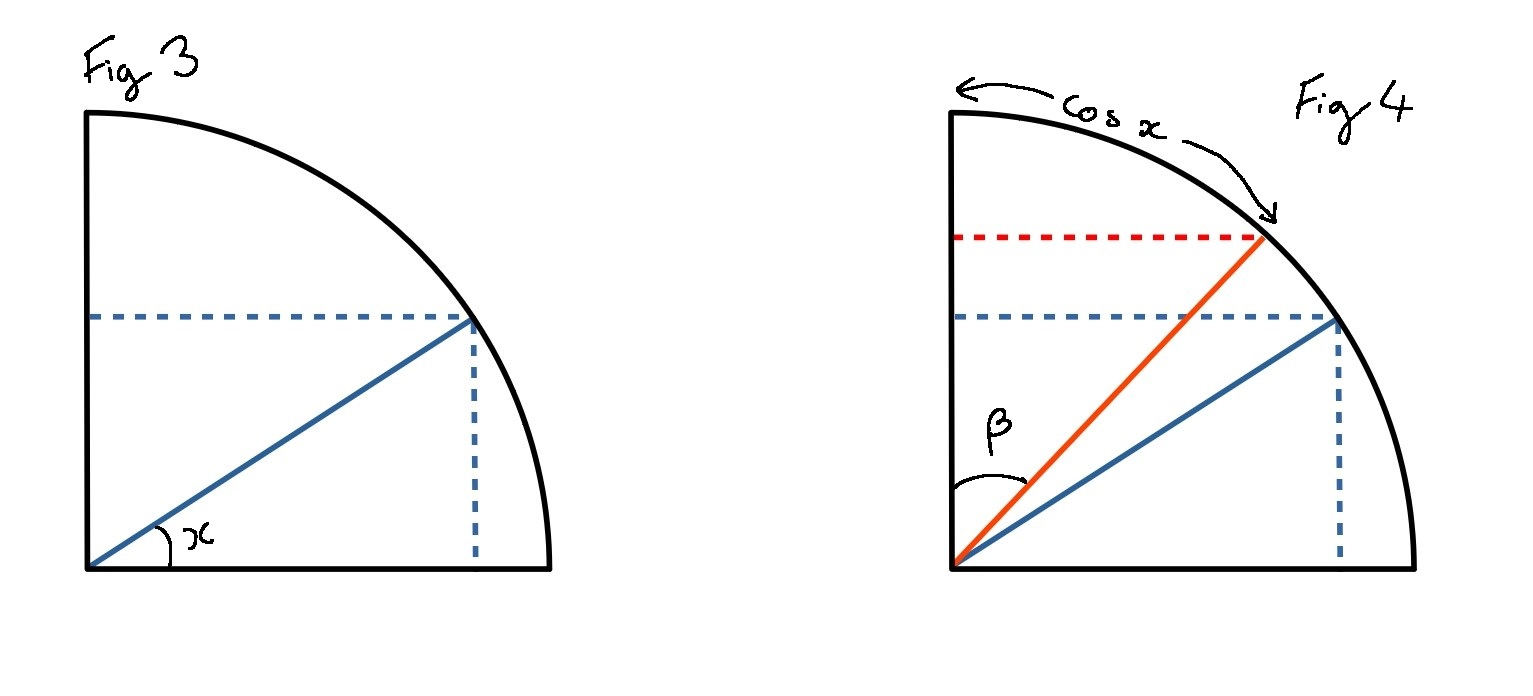#### You may also like### Shades of Fermat's Last Theorem

The familiar Pythagorean 3-4-5 triple gives one solution to (x-1)^n + x^n = (x+1)^n so what about other solutions for x an integer and n= 2, 3, 4 or 5?### Exhaustion

Find the positive integer solutions of the equation (1+1/a)(1+1/b)(1+1/c) = 2### Code to Zero

Find all 3 digit numbers such that by adding the first digit, the square of the second and the cube of the third you get the original number, for example 1 + 3^2 + 5^3 = 135.

# Making Waves

##### Age 16 to 18Challenge Level

It might be helpful to note that $1 < \frac {\pi} 2$.

From your sketches, you should be able to see that if you can prove the result for $x \in [0, \pi]$ then it will be true for all $x$.  (You might like to compare your sketches with these graphs).

You should have already shown that $\cos(\sin x) > \sin(\cos x)$ when $x=0, \frac{\pi} 2$ and $\pi$.

Can you use your sketches to help you explain why $\cos(\sin x) > \sin(\cos x)$ when $x \in \left(\frac{\pi} 2, \pi \right)$?

The argument for $x\in \left( 0, \frac{\pi} 2\right)$ is a bit harder.  One way to approach this is to try and prove the stronger result $\cos(\sin x) > \cos x > \sin(\cos x)$  for $x\in \left( 0, \frac{\pi} 2\right)$ instead.
You might like to sketch $y=\cos x$ alongside the other two graphs using Desmos to convince yourself this is true before trying to prove it!

Method 1: Calculus and increasing/decreasing functions

Lemma: $x > \sin x$ for $x\in \left( 0, \frac{\pi} 2\right)$.

• Let $f(x)=x - \sin x$
• Can you show that $f(x)$ is an increasing function?
• If $f(x)$ is an increasing function, then explain why this means that $x < \sin x$ for $x\in \left( 0, \frac{\pi} 2\right)$.

We know that $\cos x$ is a decreasing function for $x\in \left( 0, \frac{\pi} 2\right)$.  Explain why this, along with the lemma result, means that we have $\cos(\sin x) > \cos x$.

Explain why the lemma means that we have $\cos x > \sin(\cos x)$.

A lemma is an intermediate theorem in the proof of another theorem.

Method 2: Geometry

Imagine a quarter-circle where the radius is equal to $1$.• In Figure 1 explain why the arc length indicated is equal to $x$.  Find the lengths of the dotted lines in terms of $x$.  How does your diagram show that $x > \sin x$?
• In Figure 2 find $\alpha$ in terms of $x$, and also find the length of the extra dotted line.  What inequality does this give you?• Figure 3 is the same as Figure 1.  Find the lengths of the dotted lines.
• In Figure 4 find angle $\beta$ in terms of $x$. Use this and the horizontal dotted lines to prove the second half of the inequality.